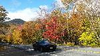# Mandelbrot SetChen Lin on 4 Oct 2021
• 25
• 291
• 6
• 0
• 248
% This is a demo created by MathWorks community team.
% Author: Ned Gulley
% Please do not vote on this demo entry.
p = 500;
x0 = -0.6;
r = 1.5;
k = 50;
x = linspace(x0-r, x0+r, p);
y = linspace(-r, r, p);
[X, Y] = meshgrid(x, y);
Z = X + 1i*Y;
m = k*ones(size(Z));
z = Z;
for n = 0:k
z = z.*z+Z;
h = abs(z)>2;
z(h) = NaN;
m(h) = n;
end
imagesc(m)
colormap([parula(k-1);0 0 0])
axis equal
axis off